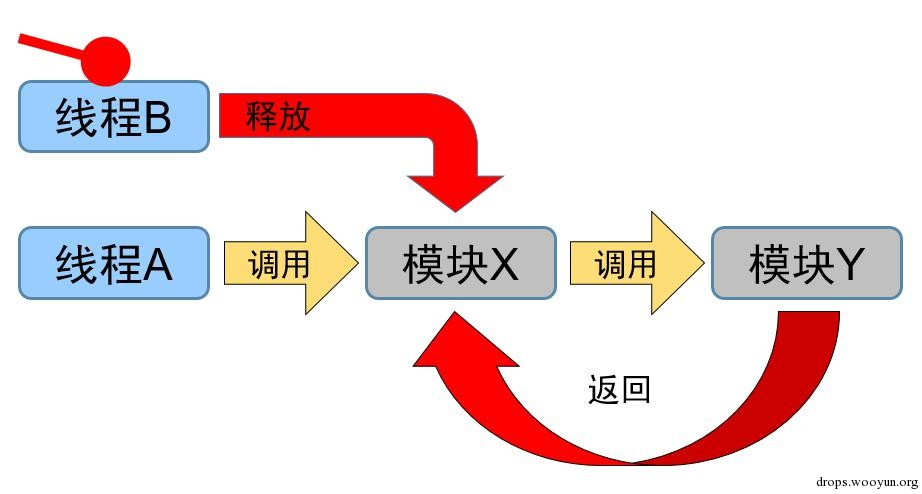Author：[email protected]

# 0x01 直接改写只读内存

IE浏览器的某些界面实际上是用HTML实现的，这些HTML通常存储在ieframe.dll的资源中，例如：打印预览是`res://ieframe.dll/preview.dlg`，整理收藏夹是`res://ieframe.dll/orgfav.dlg`，页面属性则是`res://ieframe.dll/docppg.ppg`

IE浏览器会为这些HTML创建独立的渲染实例，以及独立的JavaScript引擎实例。而为这些HTML创建的JavaScript引擎实例中，SafeMode本身就是关闭的。

``````#!bash
eax=00000041 ebx=1e2e31b0 ecx=00000000 edx=00000083 esi=1e2e31b0 edi=68b77fe5
eip=69c6585f esp=0363ac00 ebp=0363ac84 iopl=0         nv up ei pl nz na pe cy
cs=0023  ss=002b  ds=002b  es=002b  fs=0053  gs=002b             efl=00010207
jscript9!Js::JavascriptOperators::OP_SetElementI+0x117:
69c6585f 88040f          mov     byte ptr [edi+ecx],al      ds:002b:68b77fe5=76
0:008> !exchain
0363b0f0: jscript9!DListBase<CustomHeap::Page>::DListBase<CustomHeap::Page>+1570 (69b421d1)
0363b648: jscript9!DListBase<CustomHeap::Page>::DListBase<CustomHeap::Page>+1570 (69b421d1)
0363bab8: jscript9!DListBase<CustomHeap::Page>::DListBase<CustomHeap::Page>+1570 (69b421d1)
0363bb78: jscript9!DListBase<CustomHeap::Page>::DListBase<CustomHeap::Page>+28c0 (69c71564)
0363bbc0: jscript9!DListBase<CustomHeap::Page>::DListBase<CustomHeap::Page>+2898 (69c7150f)
0363bc44: jscript9!DListBase<CustomHeap::Page>::DListBase<CustomHeap::Page>+276a (69d0dedd)
0363c588: MSHTML!_except_handler4+0 (66495fa4)
CRT scope  0, filter: MSHTML! ... Omitted... (6652bbe8)
func:   MSHTML!... Omitted... (6652bbf1)
0363c62c: user32!_except_handler4+0 (7569a61e)
CRT scope  0, func:   user32!UserCallWinProcCheckWow+123 (75664456)
0363c68c: user32!_except_handler4+0 (7569a61e)
CRT scope  0, filter: user32!DispatchMessageWorker+15e (756659b7)
func:   user32!DispatchMessageWorker+171 (756659ca)
0363f9a8: ntdll!_except_handler4+0 (776a71f5)
CRT scope  0, filter: ntdll!__RtlUserThreadStart+2e (776a74d0)
0363f9c8: ntdll!FinalExceptionHandler+0 (776f7428)
``````

``````#!cpp
int __thiscall RaiseFailFastExceptionFilter(int this) {
signed int **v1; // [email protected]/* <![CDATA[ */!function(t,e,r,n,c,a,p){try{t=document.currentScript||function(){for(t=document.getElementsByTagName('script'),e=t.length;e--;)if(t[e].getAttribute('data-cfhash'))return t[e]}();if(t&#038;&#038;(c=t.previousSibling)){p=t.parentNode;if(a=c.getAttribute('data-cfemail')){for(e='',r='0x'+a.substr(0,2)|0,n=2;a.length-n;n+=2)e+='%'+('0'+('0x'+a.substr(n,2)^r).toString(16)).slice(-2);p.replaceChild(document.createTextNode(decodeURIComponent(e)),c)}p.removeChild(t)}}catch(u){}}()/* ]]&gt; */
CONTEXT *v2; // [email protected]/* <![CDATA[ */!function(t,e,r,n,c,a,p){try{t=document.currentScript||function(){for(t=document.getElementsByTagName('script'),e=t.length;e--;)if(t[e].getAttribute('data-cfhash'))return t[e]}();if(t&#038;&#038;(c=t.previousSibling)){p=t.parentNode;if(a=c.getAttribute('data-cfemail')){for(e='',r='0x'+a.substr(0,2)|0,n=2;a.length-n;n+=2)e+='%'+('0'+('0x'+a.substr(n,2)^r).toString(16)).slice(-2);p.replaceChild(document.createTextNode(decodeURIComponent(e)),c)}p.removeChild(t)}}catch(u){}}()/* ]]&gt; */
signed int v3; // [email protected]/* <![CDATA[ */!function(t,e,r,n,c,a,p){try{t=document.currentScript||function(){for(t=document.getElementsByTagName('script'),e=t.length;e--;)if(t[e].getAttribute('data-cfhash'))return t[e]}();if(t&#038;&#038;(c=t.previousSibling)){p=t.parentNode;if(a=c.getAttribute('data-cfemail')){for(e='',r='0x'+a.substr(0,2)|0,n=2;a.length-n;n+=2)e+='%'+('0'+('0x'+a.substr(n,2)^r).toString(16)).slice(-2);p.replaceChild(document.createTextNode(decodeURIComponent(e)),c)}p.removeChild(t)}}catch(u){}}()/* ]]&gt; */
UINT v4; // [email protected]/* <![CDATA[ */!function(t,e,r,n,c,a,p){try{t=document.currentScript||function(){for(t=document.getElementsByTagName('script'),e=t.length;e--;)if(t[e].getAttribute('data-cfhash'))return t[e]}();if(t&#038;&#038;(c=t.previousSibling)){p=t.parentNode;if(a=c.getAttribute('data-cfemail')){for(e='',r='0x'+a.substr(0,2)|0,n=2;a.length-n;n+=2)e+='%'+('0'+('0x'+a.substr(n,2)^r).toString(16)).slice(-2);p.replaceChild(document.createTextNode(decodeURIComponent(e)),c)}p.removeChild(t)}}catch(u){}}()/* ]]&gt; */
HANDLE v5; // [email protected]/* <![CDATA[ */!function(t,e,r,n,c,a,p){try{t=document.currentScript||function(){for(t=document.getElementsByTagName('script'),e=t.length;e--;)if(t[e].getAttribute('data-cfhash'))return t[e]}();if(t&#038;&#038;(c=t.previousSibling)){p=t.parentNode;if(a=c.getAttribute('data-cfemail')){for(e='',r='0x'+a.substr(0,2)|0,n=2;a.length-n;n+=2)e+='%'+('0'+('0x'+a.substr(n,2)^r).toString(16)).slice(-2);p.replaceChild(document.createTextNode(decodeURIComponent(e)),c)}p.removeChild(t)}}catch(u){}}()/* ]]&gt; */

v1 = (signed int **)this;
if ( !g_fFailFastHandlerDisabled )
{
v2 = *(CONTEXT **)(this + 4);
g_fFailFastHandlerDisabled = 1;
RaiseFailFastException(*(PEXCEPTION_RECORD *)this, v2, 2u);
v3 = 1653;
if ( *v1 )
v3 = **v1;
v4 = v3;
v5 = GetCurrentProcess();
TerminateProcess(v5, v4);
}
return 0;
}
``````

``````#!cpp
int result; // [email protected]/* <![CDATA[ */!function(t,e,r,n,c,a,p){try{t=document.currentScript||function(){for(t=document.getElementsByTagName('script'),e=t.length;e--;)if(t[e].getAttribute('data-cfhash'))return t[e]}();if(t&#038;&#038;(c=t.previousSibling)){p=t.parentNode;if(a=c.getAttribute('data-cfemail')){for(e='',r='0x'+a.substr(0,2)|0,n=2;a.length-n;n+=2)e+='%'+('0'+('0x'+a.substr(n,2)^r).toString(16)).slice(-2);p.replaceChild(document.createTextNode(decodeURIComponent(e)),c)}p.removeChild(t)}}catch(u){}}()/* ]]&gt; */

if ( BasepAllowResourceConversion )
else
result = 0;
return result;
}
``````

# 0x02 直接执行不可执行内存``````#!cpp
typedef struct _THUNK3 {
UCHAR MovEdx;       // 0xba         mov edx, imm32
LONG EdxImmediate;
UCHAR MovEcx;       // 0xb9         mov ecx, imm32
LONG EcxImmediate; // <- put your Stack Pivot here
USHORT JmpEcx;      // 0xe1ff       jmp ecx
} Thunk3;
``````

ATL thunk emulation机制会检查要跳往的地址是否位于PE文件中，在支持CFG的系统上还会确认要跳往的地址能否通过CFG检查。同时，在Vista之后的Windows默认 DEP policy 下，ATL thunk emulation机制仅对没有设置 IMAGE_DLLCHARACTERISTICS_NX_COMPAT的程序生效。如果程序编译时指定了/NXCOMPAT参数，就不再兼容ATL thunk emulation了。不过还是有很多程序支持ATL thunk emulation，例如很多第三方应用程序，以及32 位的 iexplore.exe。所以，类似Hacking Team泄露邮件中的CVE-2015-2425，如能用某种堆喷成功抢占内存，也可借此技巧实现漏洞利用。

（本文大部分内容完成于2014年10月，涉及的模块地址、符号信息等基于Windows Technical Preview 6.4.9841 x64 with Internet Explorer 11。）